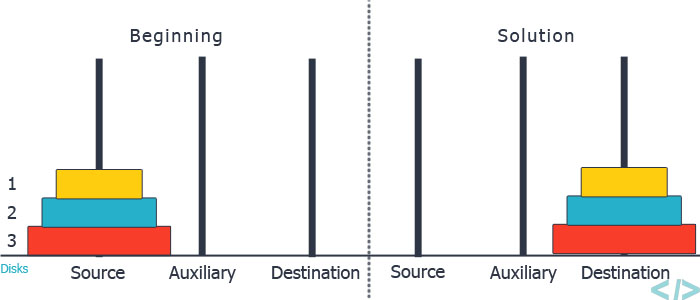30

# Tower of Hanoi

Difficulty: EASY
Avg. time to solve
15 min
Success Rate
85%

Problem Statement

#### You are given three rods (numbered 1 to 3), and ‘N’ disks initially placed on the first rod, one on top of each other in increasing order of size ( the largest disk is at the bottom). You are supposed to move the ‘N’ disks to another rod(either rod 2 or rod 3) using the following rules and in less than 2 ^ (N) moves.

``````1. You can only move one disk in one move.
2. You can not place a larger disk on top of a smaller disk.
3. You can only move the disk at the top of any rod.
``````
##### Note :
``````You may assume that initially, the size of the ‘i’th disk from the top of the stack is equal to ‘i’, i.e. the disk at the bottom has size ‘N’, the disk above that has size ‘N - 1’, and so on. The disk at the top has size 1.
``````
##### Example :##### Input Format :
``````The first line contains a single integer ‘T’ denoting the number of test cases. The test cases follow.

The first line of each test case contains a single integer ‘N’ denoting the number disks.
``````
##### Output Format :
``````For each test case, return a 2-D array/list, where each row of the array should contain exactly two integers. The first integer should be the number of the rod from where the topmost disk is to be removed and the second integer should denote the number of the rod where the removed disk is to be placed. If you have correctly moved all the disks from rod 1 to either rod 2 or rod 3 the output will be ‘1’ otherwise the output will be ‘0’.

The output of every test case will be printed in a separate line.
``````
##### Note :
``````You don’t need to print anything; It has already been taken care of.
``````
##### Constraints :
``````1 <= T <= 5
1 <= N <= 12

Where ‘T’ denotes the number of test cases, ‘N’ denotes the number of disks.

Time Limit: 1 sec
``````
##### Sample Input 1 :
``````2
1
2
``````
##### Sample Output 1 :
``````1
1
``````
##### Explanation of Sample Input 1 :
``````In the first test case,
you can move the only disk to either rod 2 or rod 3. The matrix to be returned should be either { { 1, 2 } } or { {1, 3 } }.

In the second test case,
you can move the topmost disk from rod 1 to rod 2. Then move the remaining disk from rod1 to rod 3. Now move the disk in rod 2 to rod 3. . One of the correct ways to return the output is { { 1, 2 }, { 1, 3 }, { 2, 3 } }.
``````
##### Sample Input 2 :
``````1
3
``````
##### Sample Output 2 :
``````1
``````
##### Explanation of Sample Input 2 :
``````One of the correct matrices is { { 1, 2 }, { 1, 3 }, { 2, 3 }, { 1, 2 }, { 3, 1 }, { 3, 2 }, { 1, 2 } }.
``````Console